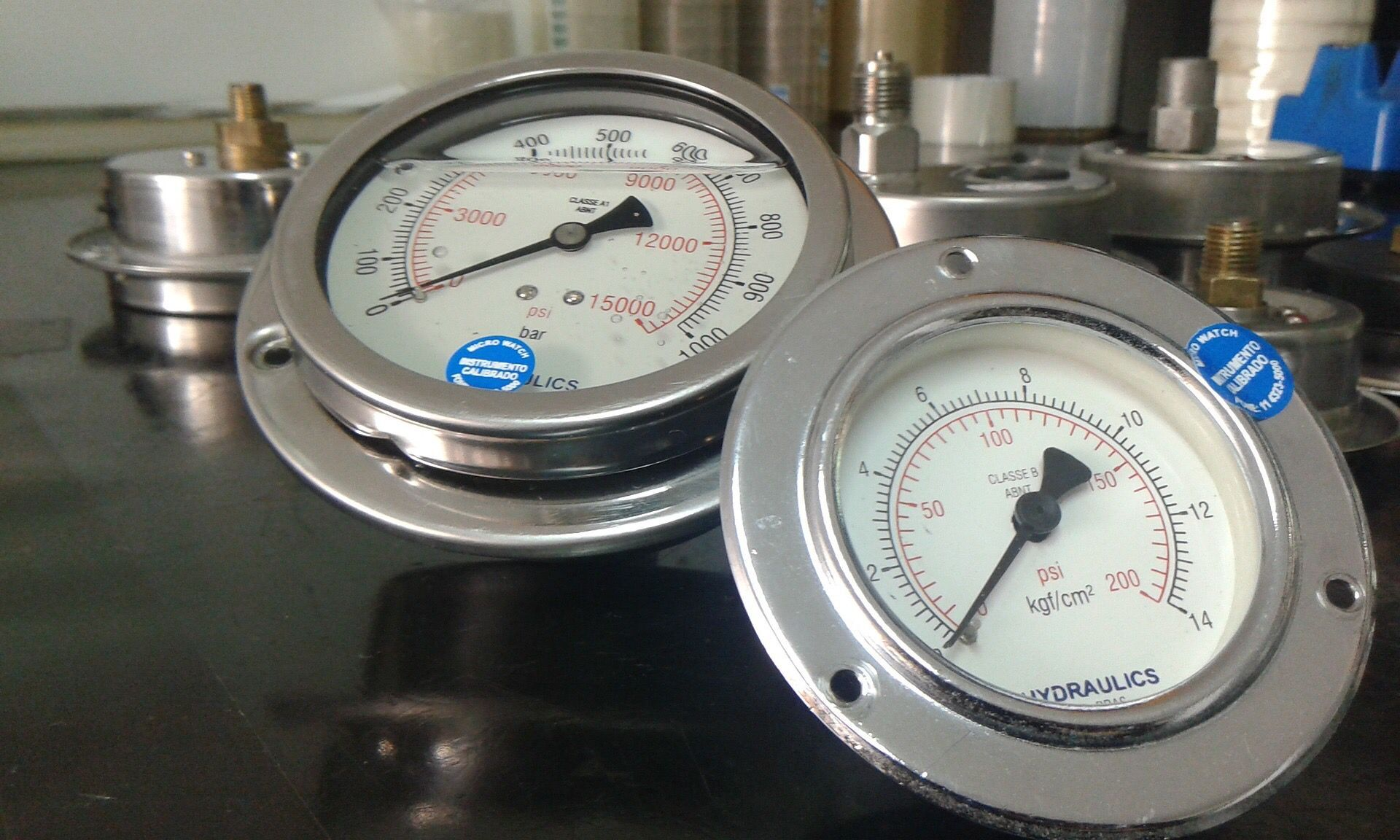# oz是什么单位（常用计量单位换算）

oz是什么单位（常用计量单位换算）

1）长度单位 公制：米(m)、厘米(cm)、毫米(mm)。 l米=100厘米=1000毫米 英制：码(yd)、英尺(ft)、英寸(in)。 1码=3英尺， 1英尺=12英寸 换算：1码 =0.9144米，1英尺 =0.3048米，l英寸=2.54厘米=25.4毫米 1米 =1.0936码 =3.2808英尺。

2）品质(重量)单位 公制：吨(t)、千克(kg)、克(g)。 1吨=1000千克，1千克=1000克，1克=1000毫克 英制：磅(lb)、盎司(oz)、格林(gr)。 1磅=16盎司=7000格林，1盎司=437.5格林换算：1磅=0.4536千克=453.6克，1盎司=28.35克，1格林=0.0648克。 1千克=2.2046磅, 1克=15.432格林，1千克=35.27盎司

3）面积单位 公制：平方米(㎡)、平方厘米(c㎡)、平方毫米(m㎡)。 1㎡=10000c㎡)，1c㎡=100m㎡ 英制：码²(yd²)换算：1米²=1.196码² 1码² = 0.8361米²

4）力的单位 公制: 牛顿(N)、厘牛顿(cN)、daN 。 1牛顿(N)=100厘牛顿(cN)，1千克力(kgf)=1000克力(gf)，1 daN=10牛顿(N)。 英制：磅力(lbf) , 盎司力(oz)换算: 1千克力(kgf)=9.807牛顿(N)， 1克力(gf)=0.9807厘牛顿(cN)， 1千克力(kgf)=2.2磅力（lbf） 1牛顿(N)=0.225 磅力(lbf), 1盎司力（oz）=28.35克力(gf)=0.06237磅力(lbf)4） 容积单位 公制：米³、升、毫升。 1米³=1000升，1升=1000毫升。

5）面密度单位 公制：千克/米²(kg/m²)，克/米²(g/m²)。 英制：盎司/码²(oz/yd²)。 换算：1千克/米²=29.4535盎司/码²

1盎司/码² =33.91克/米²